Tamilnadu Samacheer Kalvi 12th Maths Solutions Chapter 2 Complex Numbers Ex 2.9

Choose the correct or the most suitable answer from the given four alternatives:

Question 1.
in + in+1 + in+2 + in+3 is ______
(a) 0
(b) 1
(c) -1
(d) i
(a) 0

Question 2.
The value of $$\sum_{i=1}^{13}\left(i^{n}+i^{n-1}\right)$$ is ______
(a) 1 + i
(b) i
(c) 1
(d) 0
(a) 1 + i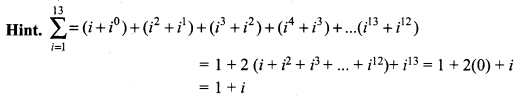Question 3.
The area of the triangle formed by the complex numbers z, iz, and z + iz in the Argand’s diagrams is ______
(a) $$\frac { 1 }{ 2 }$$ |z|
(b) |z|2
(c) $$\frac { 3 }{ 2 }$$|z|2
(d) 2|z|2
(a) $$\frac { 1 }{ 2 }$$ |z|
Hint: Area of triangle = $$\frac { 1 }{ 2 }$$ bh
= $$\frac { 1 }{ 2 }$$ |z| |iz|
= $$\frac { 1 }{ 2 }$$ |z|2

Question 4.
The conjugate of a complex number is $$\frac{1}{i-2}$$. Then, the complex number is _____
(a) $$\frac{1}{i+2}$$
(b) $$\frac{-1}{i+2}$$
(c) $$\frac{-1}{i-2}$$
(d) $$\frac{1}{i-2}$$
(b) $$\frac{-1}{i+2}$$
Hint: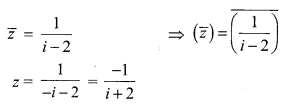Question 5.
If $$z=\frac{(\sqrt{3}+i)^{3}(3 i+4)^{2}}{(8+6 i)^{2}}$$ then |z| is equal to ______
(a) 0
(b) 1
(c) 2
(d) 3
(c) 2
Hint: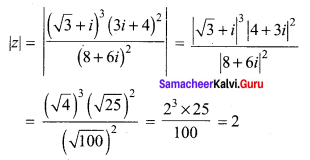Question 6.
If z is a non zero complex number, such that 2iz2 = $$\bar{z}$$ then |z| is ______
(a) $$\frac { 1 }{ 2 }$$
(b) 1
(c) 2
(d) 3
(a) $$\frac { 1 }{ 2 }$$
Hint: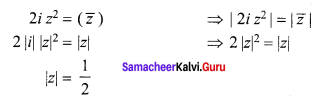Question 7.
If |z – 2 + i| ≤ 2, then the greatest value of |z| is _______
(a) √3 – 2
(b) √3 + 2
(c) √5 – 2
(d) √5 + 2
(d) √5 + 2
Hint: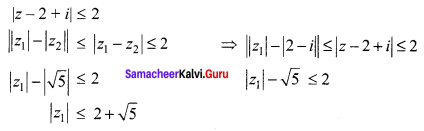Question 8.
If $$\left|z-\frac{3}{z}\right|$$, then the least value of |z| is ______
(a) 1
(b) 2
(c) 3
(d) 5
(a) 1
Hint: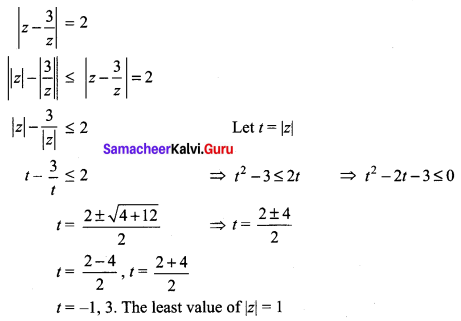Question 9.
If |z| = 1, then the value of $$\frac{1+z}{1+\bar{z}}$$ is _______
(a) z
(b) $$\bar{z}$$
(c) $$\frac { 1 }{ z }$$
(d) 1
(a) z
Hint: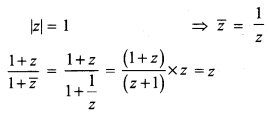Question 10.
The solution of the equation |z| – z = 1 + 2i is _______
(a) $$\frac { 3 }{ 2 }$$ – 2i
(b) –$$\frac { 3 }{ 2 }$$ + 2i
(c) 2 – $$\frac { 3 }{ 2 }$$i
(d) 2 + $$\frac { 3 }{ 2 }$$i
(a) $$\frac { 3 }{ 2 }$$ – 2i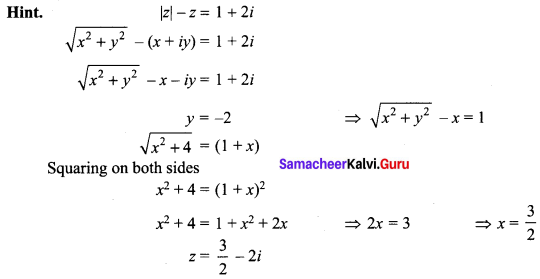Question 11.
If |z1| = 1, |z2| = 2, |z3| = 3 and |9z1 z2 + 4z1 z3 + z2 z3| = 12, then the value of |z1 + z2 + z3| is ______
(a) 1
(b) 2
(c) 3
(d) 4
(b) 2
Hint: |z1 + z2 + z3| = 2

Question 12.
If z is a complex number such that z ∈ C/R and z + $$\frac { 1 }{ z }$$ ∈ R, then |z| is ______
(a) 0
(b) 1
(c) 2
(d) 3
(b) 1
Hint: We have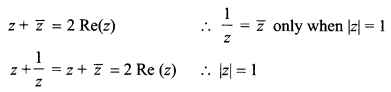Question 13.
z1, z2 and z3 are complex numbers such that z1 + z2 + z3 = 0 and |z1| = |z2| = |z3| = 1 then $$z_{1}^{2}+z_{2}^{2}+z_{3}^{2}$$ is ______
(a) 3
(b) 2
(c) 1
(d) 0
(d) 0
Hint: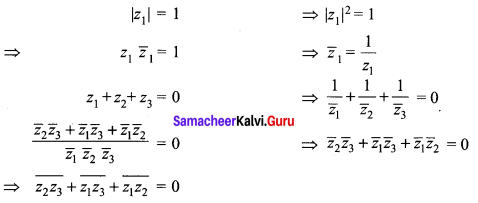Question 14.
If $$\frac{z-1}{z+1}$$ is purely imaginary, then |z| is _______
(a) $$\frac { 1 }{ 2 }$$
(b) 1
(c) 2
(d) 3
(b) 1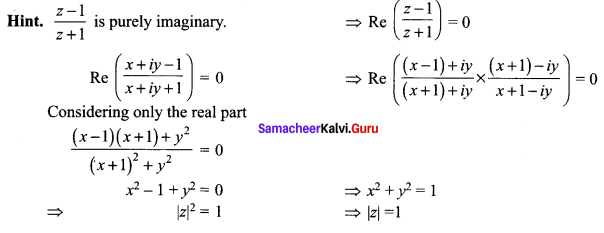Question 15.
If z = x + iy is a complex number such that |z + 2| = |z – 2|, then the locus of z is _____
(a) real axis
(b) imaginary axis
(c) ellipse
(d) circle
(b) imaginary axis
Hint:
|z + 2| = | z – 2|
⇒ |x + iy + 2| = |x + iy – 2|
⇒ |x + 2 + iy|2 = |x – 2 + iy|2
⇒ (x + 2)2 + y2 = (x – 2)2 + y2
⇒ x2 + 4 + 4x = x2 + 4 – 4x
⇒ 8x = 0
⇒ x = 0

Question 16.
The principal argument of $$\frac{3}{-1+i}$$ is ______
(a) $$-\frac{5 \pi}{6}$$
(b) $$-\frac{2 \pi}{3}$$
(c) $$-\frac{3 \pi}{4}$$
(d) $$\frac{-\pi}{2}$$
(c) $$-\frac{3 \pi}{4}$$
Hint: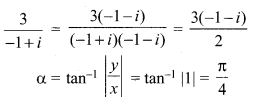The complex number lies in III quadrant. $$\theta=-(\pi-\alpha)=-\left(\pi-\frac{\pi}{4}\right)=\frac{-3 \pi}{4}$$

Question 17.
The principal argument of (sin 40° + i cos 40°)5 is ______
(a) -110°
(b) -70°
(c) 70°
(d) 110°
(a) -110°
Hint:
(sin 40° + i cos 40°)5
= (cos 50° + i sin 50°)5
= (cos 250° + i sin 250°)
To find the principal argument the rotation must be in a clockwise direction which coincides with 250°
θ = -110°

Question 18.
If (1 + i) (1 + 2i) (1 + 3i)…(1 + ni) = x + iy, then 2.5.10……(1 + n2 ) is _____
(a) 1
(b) i
(c) x2 + y2
(d) 1 + n2
(c) x2 + y2

Question 19.
If ω ≠ 1 is a cubic root of unity and (1 + ω)7 = A + B ω, then (A, B) equal to ______
(a) (1, 0)
(b) (-1, 1)
(c) (0, 1)
(d) (1, 1)
(d) (1, 1)
Hint: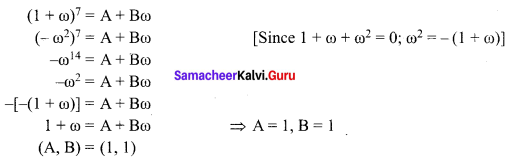Question 20.
The principal argument of the complex number $$\frac{(1+i \sqrt{3})^{2}}{4 i(1-i \sqrt{3})}$$ is _____
(a) $$\frac{2 \pi}{3}$$
(b) $$\frac{\pi}{6}$$
(c) $$\frac{5 \pi}{6}$$
(d) $$\frac{\pi}{2}$$
(d) $$\frac{\pi}{2}$$
Hint: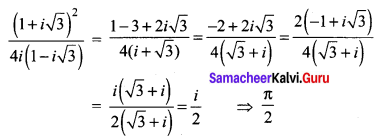Question 21.
If α and β are the roots of x2 + x + 1 = 0, then α2020 + β2020 is ______
(a) -2
(b) -1
(c) 1
(d) 2
(b) -1
Hint:
x2 + x + 1 = 0
α and β are the roots of the equation.
There are the two roots of cube roots of unity except 1.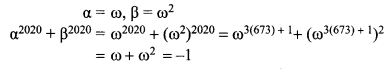Question 22.
The product of all four values of $$\left(\cos \frac{\pi}{3}+i \sin \frac{\pi}{3}\right)^{\frac{3}{4}}$$ is ____
(a) -2
(b) -1
(c) 1
(d) 2
(c) 1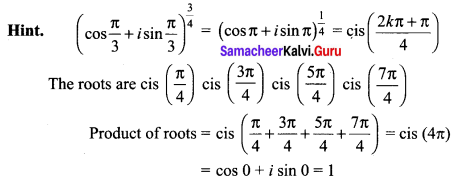Question 23.
If ω ≠ 1 is a cubic root of unity and $$\left|\begin{array}{cccc}{1} & {1} & {1} \\ {1} & {-\omega^{2}-1} & {\omega^{2}} \\ {1} & {\omega^{2}} & {\omega^{7}}\end{array}\right|$$ = 3k, then k is equal to ______
(a) 1
(b) -1
(c) i√3
(d) -i√3
(d) -i√3
Hint: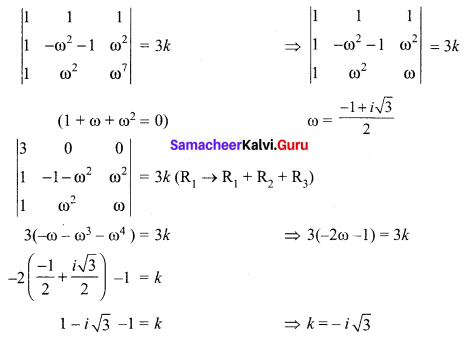Question 24.
The value of $$\left(\frac{1+\sqrt{3} i}{1-\sqrt{3} i}\right)^{10}$$ is ______
(a) cis $$\frac{2 \pi}{3}$$
(b) cis $$\frac{4 \pi}{3}$$
(c) -cis $$\frac{2 \pi}{3}$$
(d) -cis $$\frac{4 \pi}{3}$$
(a) cis $$\frac{2 \pi}{3}$$
Hint: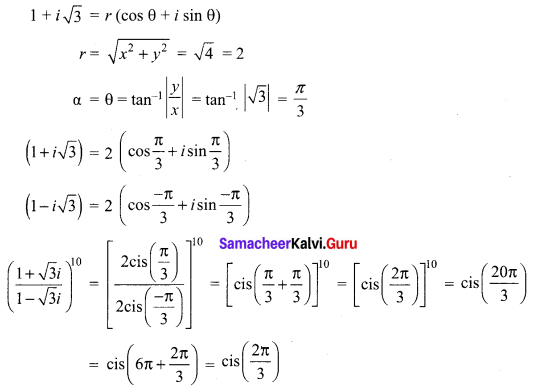Question 25.
If ω = cis $$\frac{2 \pi}{3}$$, then the number of distinct roots of $$\left|\begin{array}{ccc}{z+1} & {\omega} & {\omega^{2}} \\ {\omega} & {z+\omega^{2}} & {1} \\ {\omega^{2}} & {1} & {z+\omega}\end{array}\right|=0$$ are _____
(a) 1
(b) 2
(c) 3
(d) 4
(a) 1
Hint: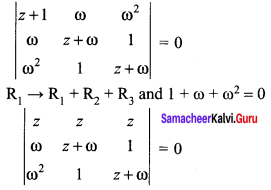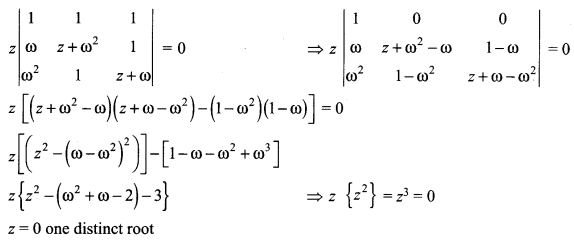Samacheer Kalvi 12th Maths Solutions Chapter 2 Complex Numbers Ex 2.9 Additional Problems

Question 1.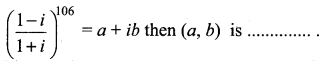(a) (2, -1)
(b) (1, 0)
(c) (0, 1)
(d) (-1, 2)
Solution:
(b) (1, 0)

Question 2.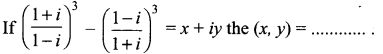(a) (0, 2)
(b) (-2, 0)
(c) (0, -2)
(d) (-2, 0)
Solution:
(c) (0, -2)

Question 3.
The point represented by the complex number 2 – i is rotated about origin through an angle
of $$\frac{\pi}{2}$$. in the clockwise direction, the new position of point is ……
(a) 1 + 2 i
(b) -1 – 2 i
(c) 2 + i
(d) -1 + 2 i
Solution:
(b) -1 – 2i
Hint.
Let z = 2 – i. Let the new position of point when the point represented by the complex number z = 2 – i is rotated about origin through an angle of $$\frac{\pi}{2}$$ in the clockwise direction be denoted by z1.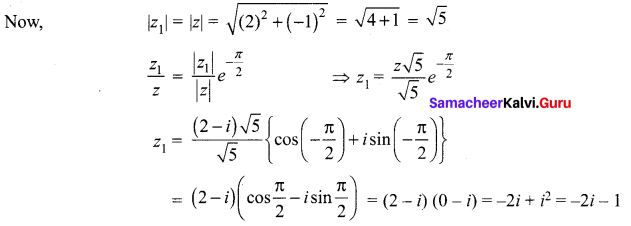Question 4.
Fill in the blanks of the following:
For any two complex numbers z1, z2 and any real number a, b,
Solution: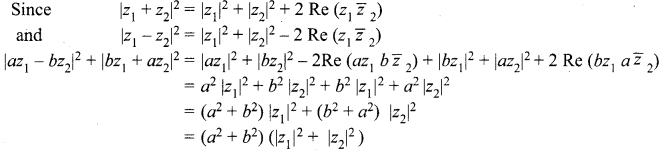Question 5.
Multiplicative inverse of 1 + i is …..
Solution: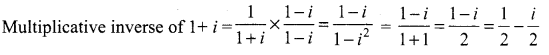Question 6.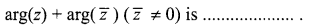Solution: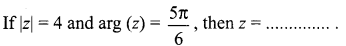Question 7.
If |z| = 4 and arg (z) = $$\frac{5 \pi}{6}$$, then z = ……
Solution: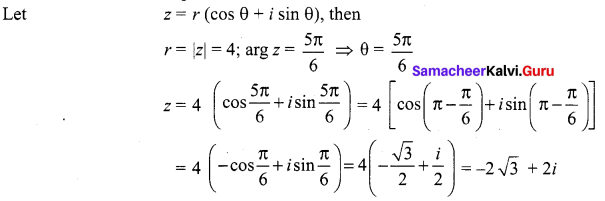Question 8.
State true or false for the following:
(i) The order relation is defined on the set of complex numbers.
Solution:
The given statement is false because the order relation “greater than” and “less than” are not defined on the set of complex numbers.

(ii) For any complex number 2, the minimum value of |z| + |z – 1| is 1.
Solution:
Let z = x + iy be any complex number.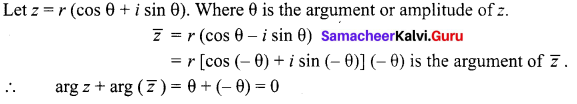When x = 0, y = 0, the value of |z| + |z – 1| is minimum and the minimum value.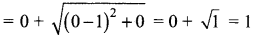Hence, the given statement is true.

(iii) 2 is not a complex number.
Solution:
It is a false statement because 2 = 2 + i(0), which is of the form α + ib, and the number of the form a + ib, where a and b are real numbers is called a complex number.

(iv) The locus represented by |z – 1| = |z – i| is a line perpendicular to the join of (1, 0) and (0, 1).
Solution:
Equation of a straight line passing through (1, 0) and (0, 1) is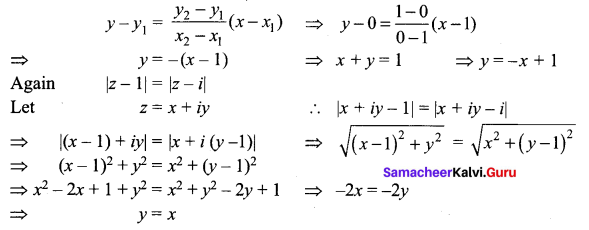Now, the lines y = -x + 1 and y = x are perpendicular to each other, so the given statement is true.

Question 9.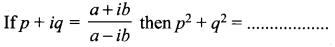Solution:
1

Question 10.
If – $$\bar{z}$$ lies in the third quadrant, then z lies in the ……..
Solution:
Hint: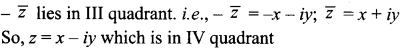Question 11.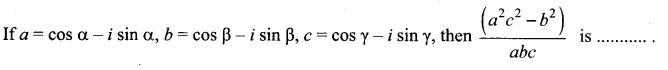(a) cos 2(α – β + γ) + i sin 2(α – β + γ)
(b) – 2cos(α – β + γ)
(c) – 2 i sin(α – β + γ)
(d) 2 cos(α – β + γ)
Solution:
(c) -2 i sin (α – β + γ)
Hint: α β γ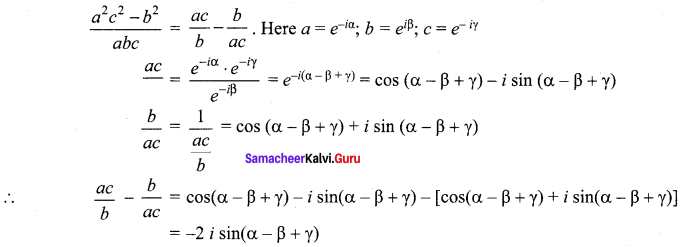Question 12.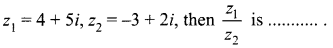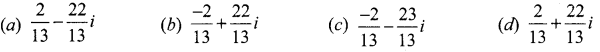Solution: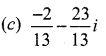Hint: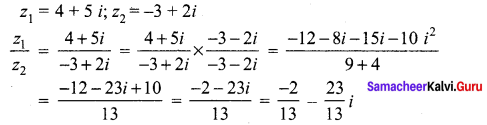Question 13.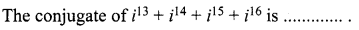(a) 1
(b) -1
(c) 0
(d)-i
Solution:
(c) 0
Hint: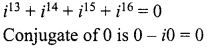Question 14.
If -i + 2 is one root of the equation ax2 – bx + c = 0, then the other root is
(a) – i – 2
(b) i – 2
(c) 2 + i
(d) 2i + i
Solution:
(c) 2 + i
Hint:
Complex roots occur in pairs when -i + 2 is one root, i.e., when 2 – i is a root, the other root is 2 + iQuestion 15.
The equation having 4 – 3i and 4 + 3i as roots is …….
(a) x2 + 8x + 25 = 0
(b) x2 + 8x – 25 = 0
(c) x2 – 8x + 25 = 0
(d) x2 – 8x – 25 = 0
Solution:
(c) x2 – 8x + 25 = 0
Hint.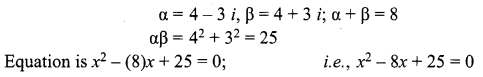Question 16.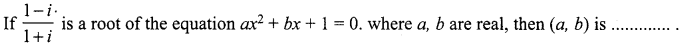(a) (1, 1)
(b) (1, -1)
(c) (0, 1)
(d) (1, 0)
Solution:
(d) (1, 0)
Hint: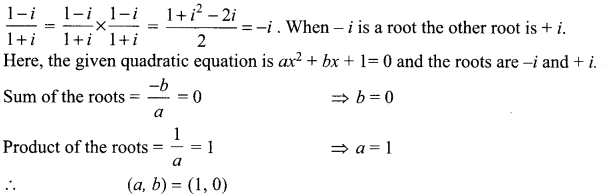Question 17.
If – i + 3 is a root of x2 – 6x + k = 0, then the value of k is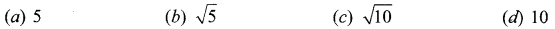Solution:
(d) 10
Hint:
α = -i + 3 = 3 – 7 ⇒ The other root is β = 3 + i
Product of the roots = αβ = (3 – i)(3 + i) = 10
Product of the roots of x2 – 6x + k = 0 is k ⇒ k = 10

Question 18.
If ω is a cube root of unity, then the value of (1 – ω + ω2)4 + (1 + ω – ω2)4 is ……
(a) 0
(b) 32
(c) -16
(d) -32
Solution:
(c) -16
Hint.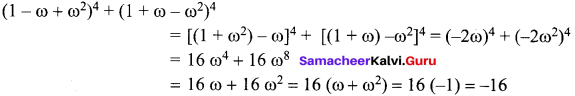Question 19.
If ω is a cube root of unity, then the value of (1 – ω) (1 – ω2) (1 – ω4)(1 – ω8) is …….
(a) 9
(b) -9
(c) 16
(d) 32
Solution:
(a) 9
Hint.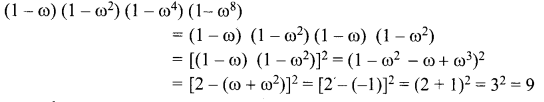Question 20.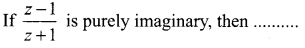(a) | z | = 1
(b) | z | > 1
(c) | z | < 1
(d) None of these.
Solution:
(a) | z | = 1